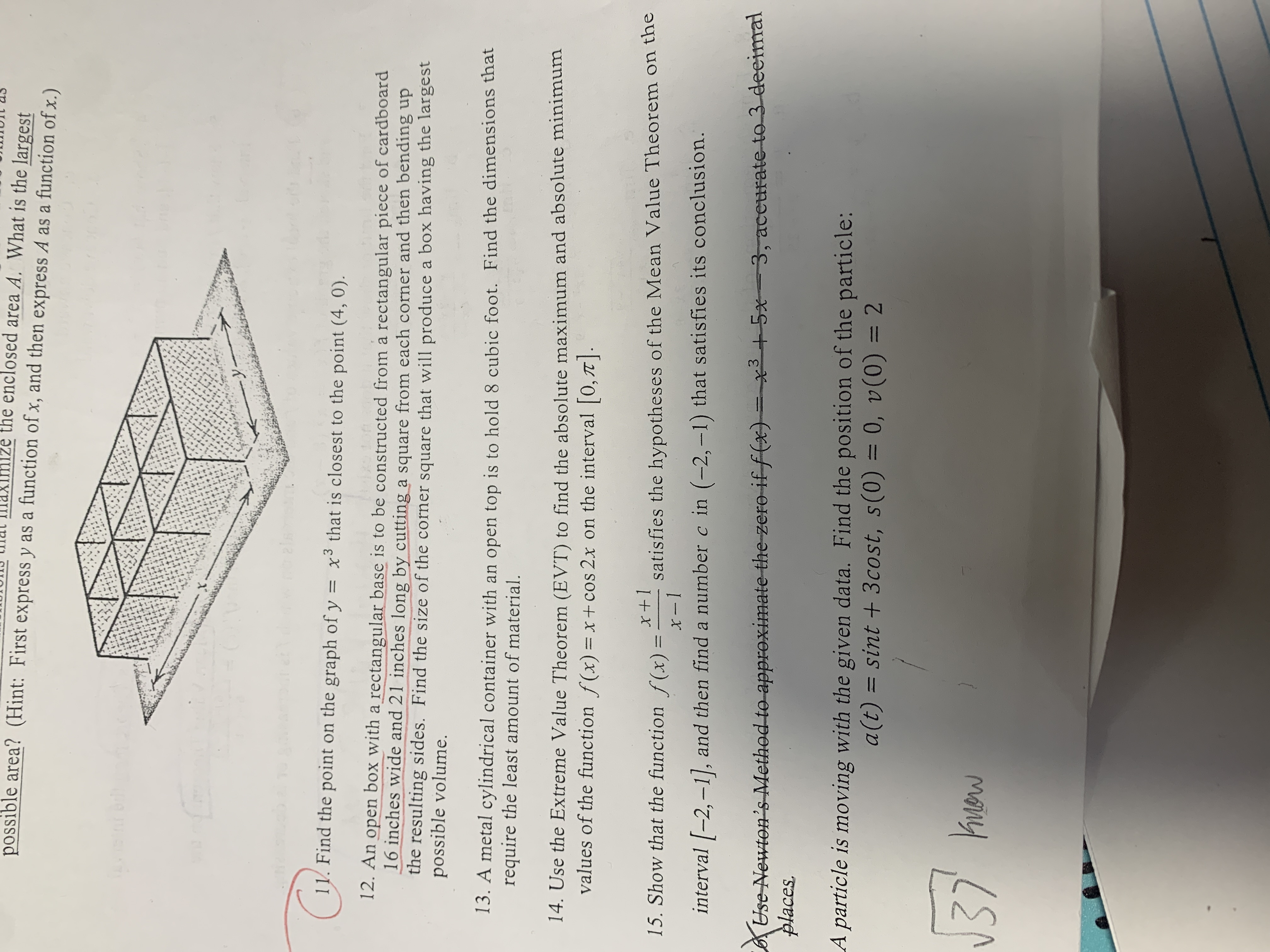# possible area? (Hint: First express y as a function of x, and then express A as a function of x.)losed area A. What is the largestthe2is11. Find the point on the graph of y x3 that is closest to the point (4, 0).12. An open box with a rectangular base is to be constructed from a rectangular piece of cardboard16 inches wide and 21 inches long by cutting a square from each corner and then bending upthe resulting sides. Find the size of the corner square that will produce a box having the largestpossible volume.13. A metal cylindrical container with an open top is to hold 8 cubic foot. Find the dimensions thatrequire the least amount of material.14. Use the Extreme Value Theorem (EVT) to find the absolute maximum and absolute minimumvalues of the function f(x)-x+ cos 2x on the interval 0,+ 1satisfies the hypotheses of the Mean Value Theorem on thex-115. Show that the function f(x) =interval -2,-1, and then find a number c in (-2,-1) that satisfies its conclusion.Use Newton's Method to approximate the-zero iffplaces.t5x3, aceurate to 3 deeimalA particle is moving with the given data. Find the position of the particle:a(t) = sint + 3cost, s(0) = 0, v(0) = 237

Question
3 views

I need help solving and understanding how to solve number 15. Thank youhelp_outlineImage Transcriptionclosepossible area? (Hint: First express y as a function of x, and then express A as a function of x.) losed area A. What is the largest the 2is 11. Find the point on the graph of y x3 that is closest to the point (4, 0). 12. An open box with a rectangular base is to be constructed from a rectangular piece of cardboard 16 inches wide and 21 inches long by cutting a square from each corner and then bending up the resulting sides. Find the size of the corner square that will produce a box having the largest possible volume. 13. A metal cylindrical container with an open top is to hold 8 cubic foot. Find the dimensions that require the least amount of material. 14. Use the Extreme Value Theorem (EVT) to find the absolute maximum and absolute minimum values of the function f(x)-x+ cos 2x on the interval 0, + 1 satisfies the hypotheses of the Mean Value Theorem on the x-1 15. Show that the function f(x) = interval -2,-1, and then find a number c in (-2,-1) that satisfies its conclusion. Use Newton's Method to approximate the-zero iff places. t5x3, aceurate to 3 deeimal A particle is moving with the given data. Find the position of the particle: a(t) = sint + 3cost, s(0) = 0, v(0) = 2 37 fullscreen
check_circle

Step 1

As per the definition, the Mean Value Theorem is defined for a point “c” between two endpoints “a” and “b” such that the tangent at the point [c,f(c)] is parallel to the secants passing through the two endpoints a and b.

Step 2

For the application of the Mean Value Theorem, the following conditions must be fulfilled.

(1) Function f must be continuous in [a,b]

(2) Function f must be differential in (a,b)

If both the condition satisfies then, there exists a single point c and the value of f '(c) can be calculated.

Step 3

First of all, checking for continuity of f(x) in [-2,-1].

We know that polynomial equations are continuous functions.

Also, (x-1) = 0 at x = 1 and 1 does not lie in (-2,-1) therefore, f(x) is continuous in [-2,-1].

To check differentiability, differentiate f(x) w.r.t. x

As (x...

### Want to see the full answer?

See Solution

#### Want to see this answer and more?

Solutions are written by subject experts who are available 24/7. Questions are typically answered within 1 hour.*

See Solution
*Response times may vary by subject and question.
Tagged in

### Limits